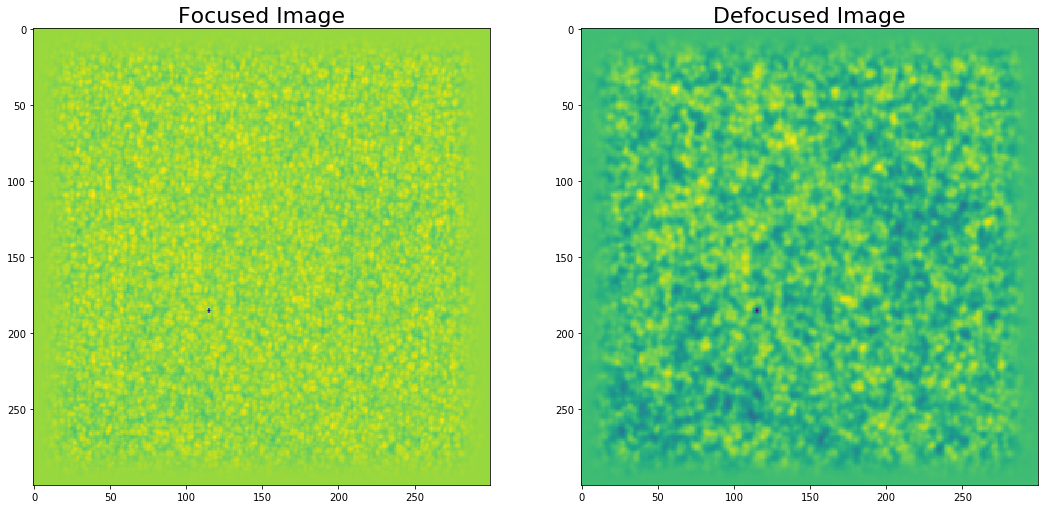# How to estimate the amount of defocus between two images

## Least square minimization to find the amout of defocus between two images¶

### It is useful sometimes for the application of phase diversity reconstruction to estimate the amount of defocus between two images. Assuming that the defocused image for the PD analysis is only defocused by changing the phase relative to the focused image and there are no other relative aberrations (e.g. plate scale change or optical misalignments), then this code could be used to estimate the amount of defocus in terms of wavelength.¶

In :
import numpy as np
import matplotlib.pyplot as plt
import scipy
from scipy.fftpack import fftshift, ifftshift, fft2, ifft2
from mpl_toolkits.axes_grid1 import make_axes_locatable, axes_size
from scipy.signal import correlate2d as correlate
from scipy.signal import general_gaussian
from astropy.io import fits
from scipy import ndimage
from functools import partial
import time
import imreg_dft
import pyfits

/home/jovyan/.local/lib/python3.7/site-packages/pyfits/__init__.py:22: PyFITSDeprecationWarning: PyFITS is deprecated, please use astropy.io.fits
PyFITSDeprecationWarning)  # noqa

In :
def pupil_size(D,lam,pix,size):
nu_cutoff = D/lam      # Cutoff frequency in rad^-1
rpupil = nu_cutoff/(2*deltanu) #pupil size in pixels
return np.int(rpupil)

def phase(coefficients,rpupil):
r = 1
x = np.linspace(-r, r, 2*rpupil)
y = np.linspace(-r, r, 2*rpupil)

[X,Y] = np.meshgrid(x,y)
R = np.sqrt(X**2+Y**2)
theta = np.arctan2(Y, X)

Z = Zernike_polar(coefficients,R,theta)
Z[R>1] = 0
return Z

def pupil_foc(coefficients,size,rpupil):
#rpupil = pupil_size(D,lam,pix,size)
A = np.zeros([size,size])
A[size//2-rpupil+1:size//2+rpupil+1,size//2-rpupil+1:size//2+rpupil+1]= phase(coefficients,rpupil)
aberr =  np.exp(1j*A)
return aberr

## function for making the aberrated phase
def phase_aberr(del_z,rpupil):
alpha_4 = (del_z*np.pi)/np.sqrt(3)
r = 1
x = np.linspace(-r, r, 2*rpupil)
y = np.linspace(-r, r, 2*rpupil)
[X,Y] = np.meshgrid(x,y)
#ph_defocus = -(np.pi*del_z*(X**2+Y**2)*D**2)/(4*lam*f**2)
R = np.sqrt(X**2 + Y**2)
ph_defocus = alpha_4  * np.sqrt(3)*(2*R**2-1)
ph_defocus[R>1] = 0
return ph_defocus

def phase_aberr_shifts(del_z,rpupil):
r = 1
x = np.linspace(-r, r, 2*rpupil)
y = np.linspace(-r, r, 2*rpupil)
[X,Y] = np.meshgrid(x,y)
ph_defocus = -(np.pi*del_z*(X**2+Y**2)*D**2)/(4*lam*f**2)
R = np.sqrt(X**2 + Y**2)
#ph_defocus = alpha_4  * np.sqrt(3)*(2*R**2-1)
ph_defocus[R>1] = 0
return ph_defocus

def savefits(data,image):
hdu = pyfits.PrimaryHDU(data)
hdu.writeto(image)

# In:

r = 1
x = np.linspace(-r, r, 2*rpupil)
y = np.linspace(-r, r, 2*rpupil)

[X,Y] = np.meshgrid(x,y)
R = np.sqrt(X**2+Y**2)
theta = np.arctan2(Y, X)
M = 1*(np.cos(theta)**2+np.sin(theta)**2)
M[R>1] = 0

## function to compute the amplitude of PSF from the complex pupil function

## making zero where the aberration is equal to 1 (the zero background)
abbe_z = np.zeros((len(abbe),len(abbe)),dtype=np.complex)
PSF = ifftshift(fft2(fftshift(abbe_z))) #from brandon
PSF = (np.abs(PSF))**2 #or PSF*PSF.conjugate()
#PSF = PSF/PSF.sum()
return PSF

#
## function to compute the OTF from PSF (to be used in PD fit )
def OTF(psf):
otf = ifftshift(psf)
otf = fft2(otf)
otf = otf/float(otf[0,0])
#sotf = otf/otf.max() # or otf_max = otf[size/2,size/2] if max is shifted to center

return otf

#
## function to return MTF from OTF:
def MTF(otf):
mtf = np.abs(otf)
return mtf

## Cosine apodization (like in JOhann's code)
def apo2d(masi,perc):
s = masi.shape
edge = 100./perc
mean = np.mean(masi)
masi = masi-mean
smooth_x = np.int(s/edge)
smooth_y = np.int(s/edge)

for i in range(0,smooth_x):

for i in range(0,s):
for i in range(0,s):
masi = masi+mean
return masi

def strehl(rms):
return np.exp(-2*(np.pi*rms**2))

def imreg(im0,imk):
import imreg_dft as ird
from image_registration import chi2_shift
from image_registration.fft_tools import shift
xoff, yoff, exoff, eyoff = chi2_shift(im0,imk)
timg = ird.transform_img(imk, tvec=np.array([-yoff,-xoff]))
return timg

def RMS_cont(data):
return data.std()/data.mean()

def noise(im):
from skimage.restoration import estimate_sigma
s = estimate_sigma(im)
return s

def RMS_error(im1,im2):
return np.sqrt(1./(im1.shape)**2 * np.sum(im1-im2)**2)

In :
p = '/home/jovyan/Targets/'

In :
d = pyfits.getdata(p+'solo_L0_phi-hrt-ilam_20200420T132834_V202004201547C_0024160022000.fits') #20 accumulations
dark = pyfits.getdata(p+'dark_kinga.fits')

flat = pyfits.getdata(p+'solo_L0_phi-hrt-ilam_20200417T234528_V202004251106C_0024150029000.fits')

/home/jovyan/.local/lib/python3.7/site-packages/pyfits/card.py:704: UserWarning: The following header keyword is invalid or follows an unrecognized non-standard convention:
RPLTSCL   [arcsec] Platescale of raw images
self._image)

In :
D = 140
lam = 617.3*10**(-6)
pix = 0.5
f = 4125.3
size = 300

rpupil = pupil_size(D,lam,pix,size)


### Let us create an artifical defocus to a subregion of the focused image loaded below¶

In :
defocus = 0.5 #defocus in wavelengths
im0 = ((d[0,:,:]/20./256.)- dark/256./20./(flat[20,:,:]/256./150. - dark/256./20.))[500:800,500:800]
size = im0.shape
A = np.zeros([size,size])
A[size//2-rpupil+1:size//2+rpupil+1,size//2-rpupil+1:size//2+rpupil+1]= phase_aberr(defocus,rpupil)# + phase_aberr_shifts(shifts,rpupil)
aberr =  np.exp(1j*A)
otf = OTF(psf)
im0 = apo2d(im0,10)
d0 = fft2(im0)
dk = d0*otf
imk = ifft2(dk).real

/opt/conda/lib/python3.7/site-packages/ipykernel_launcher.py:2: RuntimeWarning: divide by zero encountered in true_divide

/opt/conda/lib/python3.7/site-packages/ipykernel_launcher.py:99: ComplexWarning: Casting complex values to real discards the imaginary part

In :
fig, ax = plt.subplots(1,2,figsize=(18,10))

ax.imshow(im0)
ax.imshow(imk)
ax.set_title('Focused Image',fontsize=22)
ax.set_title('Defocused Image',fontsize=22)

Out:
Text(0.5, 1.0, 'Defocused Image')### and now we minimize the root mean squared error between the original focused image and an estimated one with the defocus as a free parameter¶

In :
from scipy.optimize import minimize, minimize_scalar, least_squares

def Minimize(defocus):
im0 = ((d[0,:,:]/20./256.)- dark/256./20./(flat[20,:,:]/256./150. - dark/256./20.))[500:800,500:800]
A = np.zeros([size,size])
A[size//2-rpupil+1:size//2+rpupil+1,size//2-rpupil+1:size//2+rpupil+1]= phase_aberr(defocus,rpupil)# + phase_aberr_shifts(shifts,rpupil)
aberr =  np.exp(1j*A)
otf = OTF(psf)

im0 = apo2d(im0,10)
d0 = fft2(im0)
dk = d0*otf
Imk = ifft2(dk).real
L_m = np.square(np.sum(imk-Imk))/(size*size)
return L_m

In :
p0 = [0.5]
#p0 = np.zeros(4)
mini_used = partial(Minimize)
mini = minimize(mini_used,p0,method='L-BFGS-B')

/opt/conda/lib/python3.7/site-packages/ipykernel_launcher.py:4: RuntimeWarning: divide by zero encountered in true_divide
after removing the cwd from sys.path.
/opt/conda/lib/python3.7/site-packages/ipykernel_launcher.py:99: ComplexWarning: Casting complex values to real discards the imaginary part

In :
print, mini.x

Out:
(<function print>, array([0.5]))